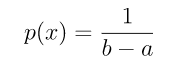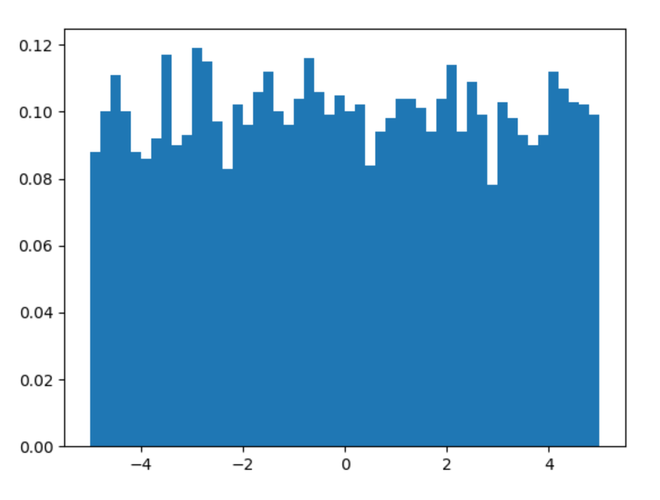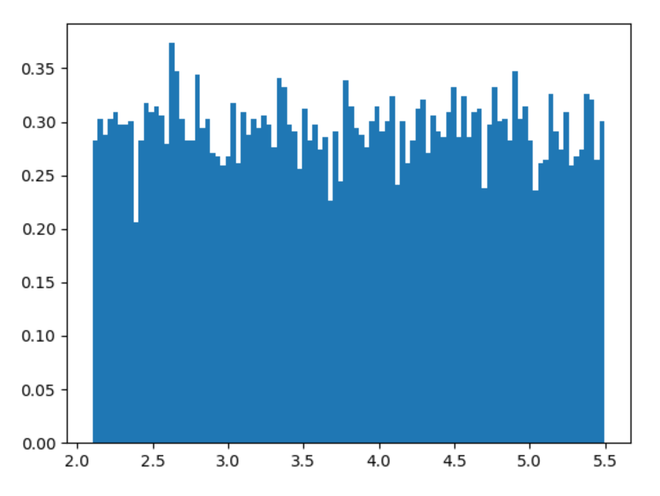GeeksforGeeks App
Open AppBrowser
Continue

# numpy.random.uniform() in Python

With the help of numpy.random.uniform() method, we can get the random samples from uniform distribution and returns the random samples as numpy array by using this method.Uniform distribution

Syntax : numpy.random.uniform(low=0.0, high=1.0, size=None)

Return : Return the random samples as numpy array.

Example #1 :

In this example we can see that by using numpy.random.uniform() method, we are able to get the random samples from uniform distribution and return the random samples.

## Python3

 `# import numpy``import` `numpy as np``import` `matplotlib.pyplot as plt`` ` `# Using uniform() method``gfg ``=` `np.random.uniform(``-``5``, ``5``, ``5000``)`` ` `plt.hist(gfg, bins ``=` `50``, density ``=` `True``)``plt.show()`

Output :Example #2 :

## Python3

 `# import numpy``import` `numpy as np``import` `matplotlib.pyplot as plt`` ` `# Using uniform() method``gfg ``=` `np.random.uniform(``2.1``, ``5.5``, ``10000``)`` ` `plt.hist(gfg, bins ``=` `100``, density ``=` `True``)``plt.show()`

Output :My Personal Notes arrow_drop_up